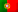# Numeral systems

In our usual day to day, we work with numbers. Everyone of us use to see numbers like 0, 1, 2, 3, 4, 5, 6, 7, 8 and 9. However, there are more numeral systems, specially those we use in computer science.

For now, it is a good practice to get used to the idea that our day to day numerical system has a name and it’s called decimal (from 10 digits from 0 to 9). And also, we have “weights” for each position: Units, tens, hundreds, thousands, etc…

In fact, we don’t have only the decimal numeral system! We have other numeral systems!

## Base-10: Decimal

Base-10 (or decimal) is our common numeral system. It may sound a little ridicule the following calculation, but it will be very useful for other numeral systems. Let’s just imagine the following number:

15943

This might look like a elementary school exercise, but let’s remember we have 3 units (weight of 1), 4 tens (weight of 10), 9 hundreds (weight of 100), 5 thousands (weight of 1000) and 1 ten thousands (weight of 10000). We should also recall that from right to left – from the units to the thousands – the “weight” grows with powers of ten. In fact, we have:

PowerDescription
100 = 1units (lower weight)
101 = 10tens
102 = 100hundreds
103 = 1000thousands
104 = 10000ten thousands (higher weight)

With the help of the following table, we could look at our number in the following way:

Ten thousandsThousandsHundredsTensUnits
104 = 10000103 = 1000102 = 100101 = 10100 = 1
15943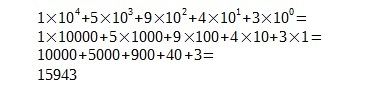It might also seem ridicule the following picture with successive divisions by 10, but just take a little look at it: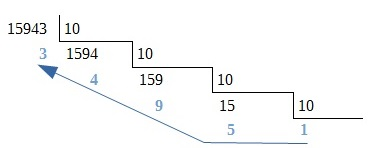If we gather the last result with all the remainders from bottom to top, we get our number back: 15943.

## Base-2 – Binary

Regarding computers, let’s now take a look on what we have. The main processor (CPU) and all logical devices that are part of a computer, work with 2 voltage levels to represent an “OFF” state, False or 0 and an “ON” state, True or 1. Therefore, we are before a binary representation since we only have 2 possible states.

Hence, the entire numeral system of a computer is limited to these 2 states: On / Off ; True / False ; 1 / 0. So, we will be restricted to two digits: 0 or 1. That’s why we say we are before a binary or base-2 numeral system.

Now, a good question! How could I represent a binary number in my decinal numeral system and vice-versa?

For example, let’s consider the following binary number:

110101

Similar to our decimal numbering, the binary digits (also known as BIT – BInary digiT), have a lower weight in the rightmost bit and a higher weight in the leftmost bit. The main difference is that the weight of each bit grows with powers of two.

Remember we have 10 digits on our decimal numeral system and that is why the weight is represented by base-10 powers. Now, with the binary numeral system we have 2 digits which means we will have powers of 2 to represent the weight of each bit.

If we build up another table, similar to the one we made in the decimal example, but now using powers of 2, we get:

MSB    LSB
25 = 3224 = 1623 = 822 = 421 = 220 = 1
110101

Performing the following calculations…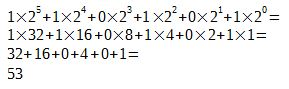… we can determine that our binary number 110101 (base-2) is equal to 53 in our decimal system (base-10).

By the way, as mentioned in the table above, we call LSB (Less Significant Bit) to the rightmost bit (lower weight) and MSB (Most Significant Bit) to the leftmost bit (higher weight).

When we work with different numeral systems we should always indicate the base as follows:

110101 (2) = 53 (10)

In the decimal numeral system, a successive division by 10 seemed to be ridicule. In fact, we would be proceeding as we were converting a number from base-10 to base-10…

But… did someone speak about converting a number from base-10 to a base-N? So, if in the previous example it seemed to be ridicule a successive division by 10, in other cases of interest we will need successive divisions by N.

So, if we want to know how to represent the decimal number 53 in binary, we only need to make the following sucessive divisions by 2.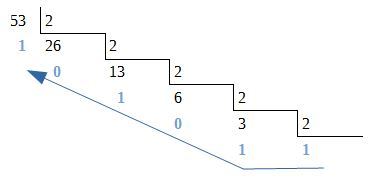In the same way, we get the last result and gather all the remainders from bottom to top.

This way, we are back to our binary number: 1 1 0 1 0 1

It is not an easy task to work directly with binary numbers, especially if we go back in time to the “stone age” of computer science. In that time, almost every programs were written in machine code and the need to have a way to represent sets of binary numbers by a more “human readable” numbering, gave birth to other numeral systems like octal and hexadecimal.

## Base 8 – Octal

Let’s talk a little about the octal numeral system. As its name suggests, it’s a system formed by 8 digits from 0 to 7. Analogously to the binary system, we might conclude that the weight of each of these numbers is related with powers of 8.

Let’s consider the following octal number:

65

And let’s create another table, this time with powers of 8:

 81 = 8 80 = 1 6 5

Easily we get: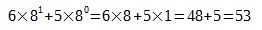This means we have:

65(8) = 53(10)

Now, to convert from decimal to octal, we will use again the method of the successive divisions, this time by 8.

I’m not going to represent the calculations as I did before hence we can easily can find the answer: 53 ÷ 8 is 6 and the remainder is 5. So, we have 65 in the octal numeral system.

Now, if we pay more attention, we know that 3 bits have 8 possible combinations (23 = 8). Therefore we can easily conclude that each octal number can be represented by a set of 3 bits. In this case, the conversion between octal and binary is a piece of cake.

Let’s take another look at the octal number 65 and let’s convert each digit to binary by making sucessive divisions by 2:Because each octal number has a weight of powers of 8, just like each set of 3 bits, we can group the binary results in the same way:

1 1 0 (6) and 1 0 1 (5), and that means we have our already known binary number as 1 1 0 1 0 1

So:

65(8) = 110101(2) = 53(10)

Making the inverse operation it’s also easy if we follow the same principle of having sets of 3 bits:

 22 = 4 21 = 2 20 = 1 22 = 4 21 = 2 20 = 1 1 1 0 1 0 1

Then we shall have, for each set, the following calculations: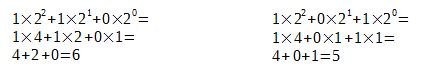This is:

110101(2) = 65(8) = 53(10)

Meantime, the octal numeral system is becoming less useful in computer science. Nowadays, when we work with binary numbers, we work with multiples of 8 bits (where each set of 8 bits represents the so called byte). If we separate each set of 8 bits on smaller sets of 4 bits, we will have 24 = 16 possible combinations. Hence, the welcome to the hexadecimal numeral system or base-16!

In the haxadecimal numeral system we have 16 digits formed by the numbers from 0 to 9 and the letters from A to F. The following table shows the relations between base-2, base-10 and base-16.

000000
000111
001022
001133
010044
010155
011066
011177
100088
100199
101010A
101111B
110012C
110113D
111014E
111115F

From this table, we can get some interesting things:

• For hexadecimal numbers, the letters represent: A = 10; B = 11; C = 12; D = 13; E = 14 and F = 15.
• In a binary number is extremely easy to check if it represents an odd or even number. If we just look at the LSB (Less Significant Bit – the rightmost bit), we see that all odd numbers have their LSB set to 1 and all even numbers have their LSB set to 0..
• If we take a better look at the binary numbers, we can understand how we can count them and how they take a 1 from right to left. It’s easy: With just 1 bit we have 2 combinations (21 = 2) – 0 or 1; With 2 bits we have 4 combinations (22 = 4) – 00, 01, 10 or 11; And so on…

Let’s now return  to conversions and let’s see how we can convert from hexadecimal to decimal.

Let’s see the following number:

8A1D

Remember that:

• A has the value of 10
• D has the value of 13
• Note: This hexadecimal number can also be represented by 841Dh or 0x841D

Again, let’s check the famous table, now with powers of 16:

 163 = 4096 162 = 256 161 = 16 160 = 1 8 A 1 D

We will have the following calculations:

8A1D(16) = 35357(10) (or 8A1Dh = 35357)

Let’s now perform successive divisions by 16 to convert from decimal to hexadecimal: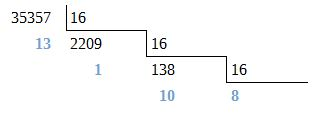We will get:

35357(10) = 8A1D(16) (ou 35357 = 8A1Dh)

To end with, just check out how easy is to convert between hexadecimal and binary!

 8 A 1 D 1000 1010 0001 1101

This time I didn’t make the calculations but with the help of the table with the representation of these values, the reader should easily reach these results by now. Could we do this with octal numbers too? Sure:

 (00) 1 0 0 0 1 0 1 0 0 0 0 1 1 1 0 1 1 0 5 0 3 5

Finally, we have the following values for each base:

1000 1010 0001 1101 (2) = 105035 (8) = 35357 (10) = 8A1D (16)

HISTORICAL NOTE: Base-8 (octal)

Readers could be trying to understand why an octal numeral system was so often used in computer science. We learned that an octal number can be converted to binary in sets of 3 bits since 23 = 8. Therefore, a set of 6 bits could be easily represented by 2 octal numbers and vice-versa.

To get the answer to this question, we need to go back in time to the very begining of the computer science. While today one byte is represented by 8 bits (and therefore why we use the hexadeciamal system to represent each set of 4 bits), in the past one byte was represented by… 6 bits!